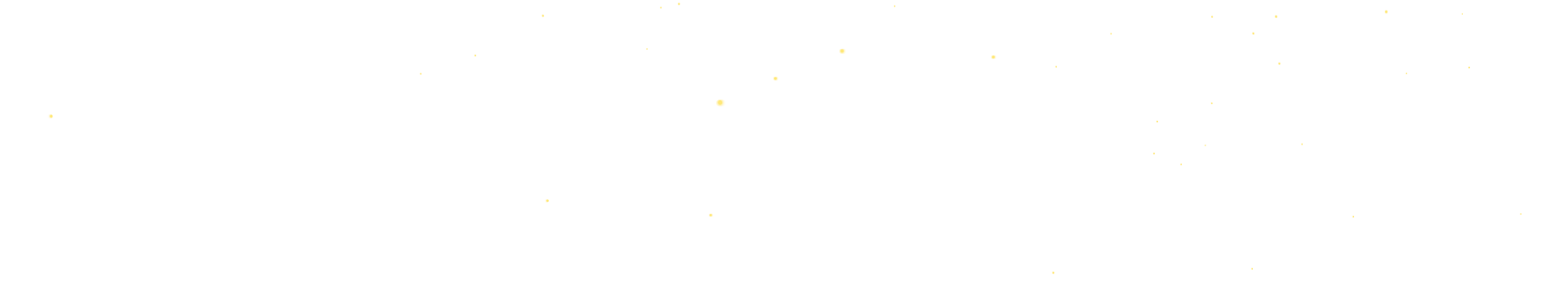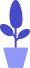# Python Pygame drawing functions

Estimated reading: 7 minutes 59 views

Like turtle drawing, Pygame has various functions for drawing shapes. These shapes include rectangles, circles, ellipses, lines, or individual pixels, and are often referred to as drawing primitives. In this tutorial, let’s learn some common plotting functions.

## pygame.draw.line() function

Let’s first look at the function pygame.draw.line() for drawing line segments, which has 5 parameters, namely:

• the surface object to be displayed;
• the color of the brush;
• starting position coordinates;
• end position coordinates;
• Line thickness.

Combined with the content introduced earlier, let’s write a simple program that uses 3 straight lines to form a letter H.

• First, create a Pygame program window;
• Then 3 variables are created to hold the tuple of colors, the 3 colors used are black, white and red;
• Next, fill the created Surface object with white, that is, set the background color of the window to white, see the “Tips” section below for details;
• Then, draw 3 lines of different colors on the Surface object, the width of the lines is 2 pixels, and call the pygame.display.update() function to display all the contents of the Surface object to the window;
• Finally, through the event loop, monitor user actions. When the close button is clicked, the window is closed.

Tip: We use capital letters to define these three color variables – BLACK, WHITE and RED, to show that the content of these three variables will not be easily changed, which is a conventional way.The complete code is as follows.

``````import pygame
# create pygame
pygame.init()
# create window
windowSurface=pygame.display.set_mode([500,400])
# create color variable
BLACK=(0,0,0)
WHITE=(255,255,255)
RED=(255,0,0)
GREEN=(0,255,0)
BLUE=(0,0,255)
# Fill the Surface object with white
windowSurface.fill(WHITE)
# draw line segments on the surface object
pygame.draw.line(windowSurface, BLACK, (60, 60), (60, 120), 2)
pygame.draw.line(windowSurface, RED, (60, 90), (90, 90), 2)
pygame.draw.line(windowSurface, BLACK, (90, 60), (90, 120), 2)
# Display the contents of the surface object to the window
pygame.display.update()
# run the event loop
Running=True
while Running:
for event in pygame.event.get():
if event.type == pygame.QUIT:
Running =False
pygame.quit()``````

Running this code, we can see a Pygame window with 3 straight lines forming a letter H, as shown in Figure 1.figure 1
For this program, we want to fill the entire Surface object stored in the windowSurface variable with white. The fill() function will fill the entire Surface object with the color passed to the parameter.

In pygame, the window on the screen does not change when the fill() method or any other drawing function is called. Instead, these functions will change the Surface object, but will not draw the new Surface object to the screen until the pygame.display.update() function is called.

This is because it is faster to modify the Surface object in computer memory than to modify the image on the screen. It is much more efficient to draw on the screen after all drawing functions have finished drawing the Surface object.

## pygame.draw.rect() function

pygame.draw.rect() is a function that draws a rectangle. It has 4 parameters, namely:

• Surface object to be displayed;
• the color of the brush;
• A tuple of 4 integers (representing the X and Y coordinates of the upper left corner, and the width and height, respectively);
• Line thickness, if it is 0, it means the rectangle is filled.

If you use this function to draw a rectangle with a red border, the code is as follows.

``````import pygame
# create pygame
pygame.init()
# create window
windowSurface=pygame.display.set_mode([500,400])
# create color variable
BLACK=(0,0,0)
WHITE=(255,255,255)
RED=(255,0,0)
GREEN=(0,255,0)
BLUE=(0,0,255)
# Fill the Surface object with white
windowSurface.fill(WHITE)
#Draw a rectangle with a red border
pygame.draw.rect(windowSurface, RED, (60, 200, 200, 100), 1)
# Display the contents of the surface object to the window
pygame.display.update()
# run the event loop
Running=True
while Running:
for event in pygame.event.get():
if event.type == pygame.QUIT:
Running =False
pygame.quit()``````

It can be seen that the method of calling the pygame.draw.rect() function is similar to the method of calling the pygame.draw.line() function, and the drawn graph is shown in Figure 2.figure 2

## pygame.draw.circle() function

pygame.draw.circle() is a function that draws a circle. It has 5 parameters, namely:

• Surface object to be displayed;
• the color of the brush;
• The coordinates of the center of the circle to be drawn;
• The radius of the circle to be drawn;
• Line thickness, if it is 0, it means the circle is filled.

If you use this function to draw a solid black circle with a radius of 20, the code is as follows:

``````import pygame
# create pygame
pygame.init()
# create window
windowSurface=pygame.display.set_mode([500,400])
# create color variable
BLACK=(0,0,0)
WHITE=(255,255,255)
RED=(255,0,0)
GREEN=(0,255,0)
BLUE=(0,0,255)
# Fill the Surface object with white
windowSurface.fill(WHITE)
#Draw a solid black circle with a radius of 20
pygame.draw.circle(windowSurface, BLACK, (350, 75), 40, 0)
# Display the contents of the surface object to the window
pygame.display.update()
# run the event loop
Running=True
while Running:
for event in pygame.event.get():
if event.type == pygame.QUIT:
Running =False
pygame.quit()``````

The drawn graph is shown in Figure 3.image 3

## pygame.draw.polygon() function

pygame.draw.polygon() is a function for drawing polygons. It has 4 parameters, namely:

• Surface object to be displayed;
• the color of the brush;
• A list of coordinates, the multiple sides of the polygon are formed by drawing lines between each coordinate point and its subsequent coordinate points in turn, and then drawing lines from the last point to the first point;
• Line thickness, if it is 0 or not filled, it means the polygon is filled.

If you use this function to draw a green filled pentagon, the code is as follows:

``````import pygame
# create pygame
pygame.init()
# create window
windowSurface=pygame.display.set_mode([500,400])
# create color variable
BLACK=(0,0,0)
WHITE=(255,255,255)
RED=(255,0,0)
GREEN=(0,255,0)
BLUE=(0,0,255)
# Fill the Surface object with white
windowSurface.fill(WHITE)
# draw a green filled pentagon
pygame.draw.polygon(windowSurface, GREEN, ((300, 100), (445, 206),(391, 377), (210, 377), (154, 206)))
# Display the contents of the surface object to the window
pygame.display.update()
# run the event loop
Running=True
while Running:
for event in pygame.event.get():
if event.type == pygame.QUIT:
Running =False
pygame.quit()``````

The drawn graph is shown in Figure 4.Figure 4

## pygame.draw.ellipse() function

The pygame.draw.ellipse() function draws an ellipse, which has 4 parameters, namely:

• Surface object to be displayed;
• the color of the brush;
• The bounding rectangle is the smallest rectangle that can be drawn around this shape. The parameter can be a pygame.Rect object or a tuple of 4 integers;
• Line thickness.

If you use this function to draw a blue ellipse, the code is as follows

``````import pygame
# create pygame
pygame.init()
# create window
windowSurface=pygame.display.set_mode([500,400])
# create color variable
BLACK=(0,0,0)
WHITE=(255,255,255)
RED=(255,0,0)
GREEN=(0,255,0)
BLUE=(0,0,255)
# Fill the Surface object with white
windowSurface.fill(WHITE)
#make a blue oval
pygame.draw.ellipse(windowSurface, BLUE, (300, 250, 40, 80), 1)
# Display the contents of the surface object to the window
pygame.display.update()
# run the event loop
Running=True
while Running:
for event in pygame.event.get():
if event.type == pygame.QUIT:
Running =False
pygame.quit()``````

The drawn graph is shown in Figure 5.Figure 5
Let’s put these drawing functions together to see the drawing effect. The code is as follows.

``````#import the pygame module
import pygame
# create pygame
pygame.init()
# create window
windowSurface=pygame.display.set_mode((500,400))
# create color constants
BLACK=(0,0,0)
WHITE=(255,255,255)
RED=(255,0,0)
GREEN=(0,255,0)
BLUE=(0,0,255)
# Fill the Surface object with white
windowSurface.fill(WHITE)
# Draw line segments on the Surface object
pygame.draw.line(windowSurface, BLACK, (60, 60), (60, 120), 2)
pygame.draw.line(windowSurface, RED, (60, 90), (90, 90), 2)
pygame.draw.line(windowSurface, BLACK, (90, 60), (90, 120), 2)
# Draw the circle on the Surface object
pygame.draw.circle(windowSurface, BLACK, (350, 75), 40, 0)
# Draw polygons on the Surface object
pygame.draw.polygon(windowSurface, RED, ((300, 100), (445, 206),(391, 377), (210, 377), (154, 206)))
# Draw rectangle on Surface object
pygame.draw.rect(windowSurface, RED, (60, 200, 200, 100), 1)
# Draw an ellipse on the Surface object
pygame.draw.ellipse(windowSurface, BLACK, (300, 250, 40, 80), 1)
# Display the contents of the Surface object to the window
pygame.display.update()
# game loop
Running=True
while Running:
# event loop
for event in pygame.event.get():
if event.type == pygame.QUIT:
Running=False
pygame.quit()``````

Run the code and the result is shown in Figure 6.